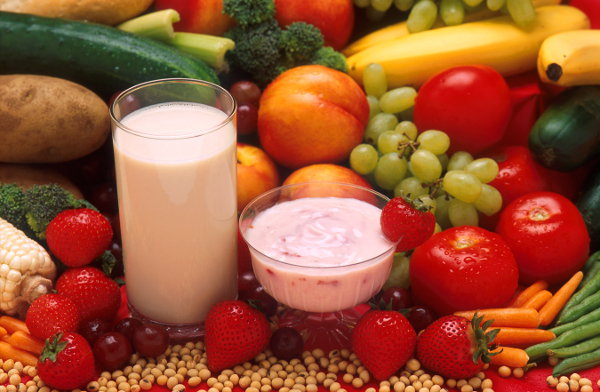# Multiply Decimals

## Multiplying Decimals by Powers of Ten:

If you multiply a decimal number by 10, the decimal point moves 1 place to make the number larger
(for example 3.456 × 10 = 34.56).

If you multiply a decimal number by 100, the decimal point moves 2 places to make the number larger
(for example 3.456 × 100 = 345.6).

If you multiply a decimal number by 1000, the decimal point moves 3 places to make the number larger
(for example 3.456 × 1000 = 3456).

## Dividing Decimals by Powers of Ten:

If you divide a decimal number by 10, the decimal point moves 1 place to make the number smaller
(for example 2345.6 ÷ 10 = 234.56).

If you divide a decimal number by 100, the decimal point moves 2 places to make the number smaller
(for example 2345.6 ÷ 100 = 23.456).

If you divide a decimal number by 100, the decimal point moves 3 places to make the number smaller
(for example 2345.6 ÷ 1000 = 2.3456).

Q1. 57.8 × 100
Q2. 45.9 × 1000
Q3. 0.087 × 10
Q4. 569.3 ÷ 10
Q5. 0.078 ÷ 100

A1. 5780
A2. 45900
A3. 0.87
A4. 56.93
A5. 0.00078

## Multiplying Decimals by Other Numbers:

Jesse J's latest album (with 17 tracks) can be downloaded as iTunes at \$0.15 per track. How much does it cost to download the whole album?

(Multiply these numbers as if they had no decimal point first. When multiplying decimals, don't line up the columns. Then place the decimal point back at the end. The total number of decimal places that you start with is the total number of decimal places that will be in the final answer.)

 \$ 0.15 × 17 \$ 2.55

## Example Two - Fuel Consumption

An old (but very cool) Porsche 911 car has a fuel consumption of 6.7 litres per 100 kilometres. (This means that for every 100 km it travels, it uses 6.7 litres of fuel.) If fuel costs 198 cents per litre, what is the fuel cost to travel 1000 kilometres from Berlin to Paris?

1000 ÷ 100 × 6.7 × \$1.98 = \$132.66

## Questions - Food EnergyNUMBER OF CALORIES IN SOME COMMON FOODS Apple = 70 Milk (1 cup) = 148 Potato chips (10 chips) = 240 Banana = 97 Malted milk (1 cup) = 276 Steamed rice (1 cup) = 120 Carrot = 28 Yogurt (1 cup) = 237 Fried rice (1 cup) = 267 To convert calories to kilojoules, multiply by 4.2

Refer to the food energy table above. What is the number of kilojoules of energy in:
(a) 1 cup of milk and 1 banana
(b) 1 steamed rice and 1 malted milk?